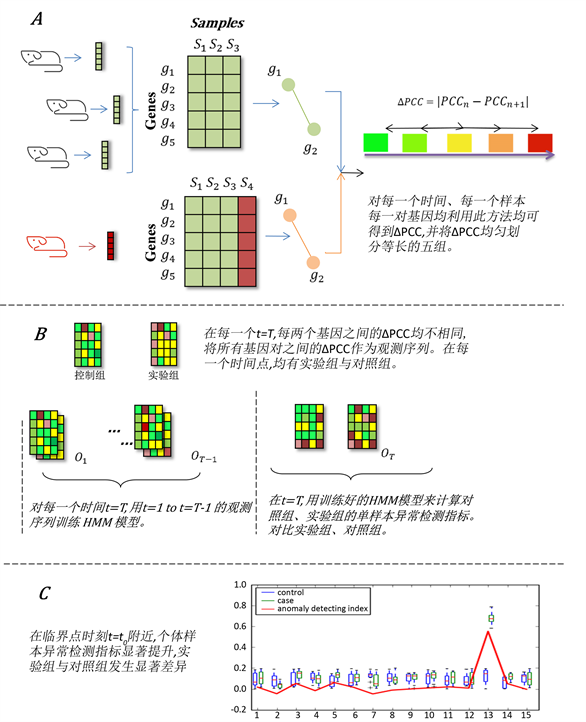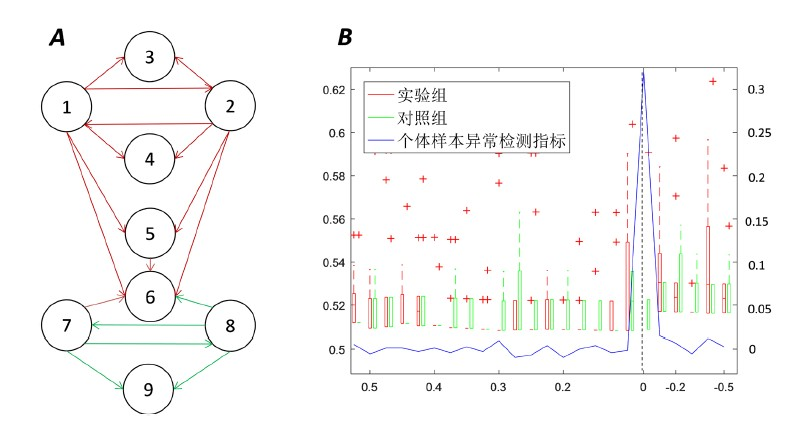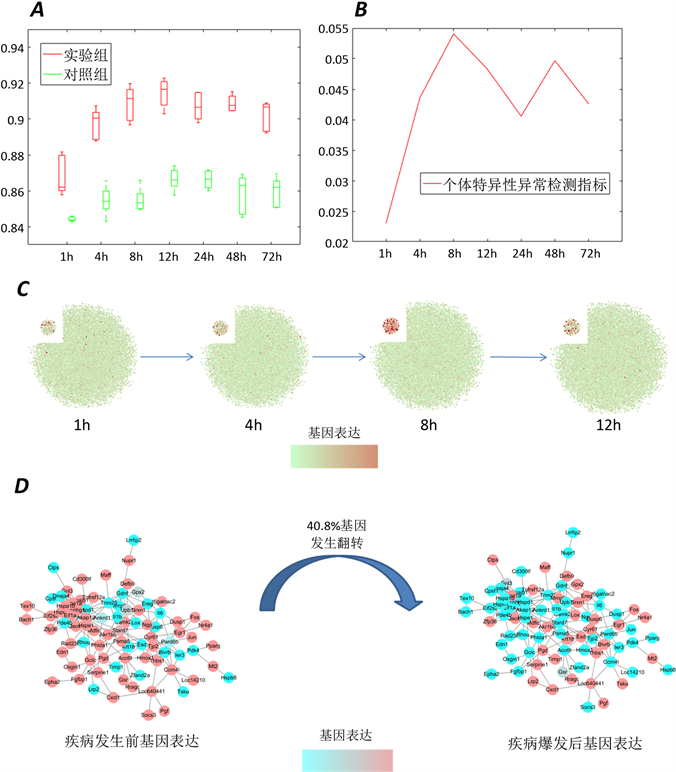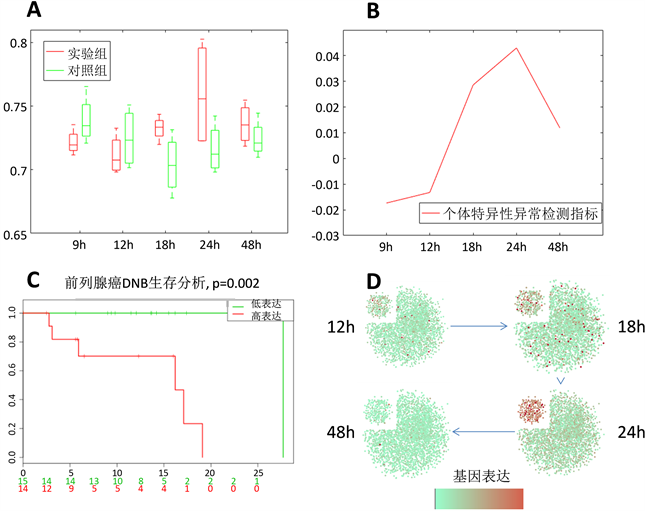# 构建个体特异性异常指标探测复杂疾病网络临界点 Detecting Critical Point of Complex Disease Network by Constructing Individual-Specific Anomaly Index

• 全文下载: PDF(3482KB)    PP.59-69   DOI: 10.12677/HJCB.2018.84008
• 下载量: 193  浏览量: 680

Detecting critical points of complex diseases is very important for early diagnosis of diseases. By exploring information of high-throughput data, we combine individual-specific network and hidden Markov model to construct individual-specific abnormal indicators in order to detect the critical points between relative health period and disease critical period. To verify the validity of the method, it was applied to simulated data sets, lung acute injury data and prostate cancer data. The critical points were successfully found before malignant mutation. The validity and sensitivity of signal genes were verified by biological function analysis.

1. 引言

2. 方法

2.1. 单样本个性化网络

${\mu }_{\Delta PC{C}_{i}}=0$ , ${\sigma }_{\Delta PC{C}_{i}}=\frac{1}{N-1}\left(1-PC{C}_{i}^{2}\right)$ (1)

${z}_{i}=\frac{\Delta PC{C}_{i}}{\left(1-PC{C}_{i}\right)/\left(n-1\right)}$ (2)

2.2. 隐马尔科夫模型

Table 1. Variable setting of hidden Markov model

$P\left(t\right)={P}_{t}\left({s}_{t}={w}_{2}|{s}_{t-1}={w}_{1},{s}_{t-2}={w}_{1},\cdots ,{s}_{1}={w}_{1},{\theta }_{t-1},O\right)$ (3)

$I\left(t\right)=1-{P}_{t}\left({s}_{t}={w}_{1}|{s}_{t-1}={w}_{1},{s}_{t-2}={w}_{1},\cdots ,{s}_{1}={w}_{1},{\theta }_{t-1},O\right)$ (4)

$\begin{array}{c}I\left(t\right)=1-{P}_{t}\left({s}_{t}={w}_{1}|{s}_{t-1}={w}_{1},{\theta }_{t-1},O\right)\\ =1-\frac{{P}_{t}\left({s}_{t}={w}_{1},{s}_{t-1}={w}_{1}|{\theta }_{t-1},O\right)}{{P}_{t}\left({s}_{t-1}={w}_{1}|{\theta }_{t-1},O\right)}\end{array}$ (5)

2.3. 算法设计

${I}_{j}\left(T\right)=1-\frac{{P}_{t}\left({s}_{t}={w}_{1},{s}_{t-1}={w}_{1}|{\theta }_{t-1},{o}_{j}^{T}\right)}{{P}_{t}\left({s}_{t-1}={w}_{1}|{\theta }_{t-1},{o}_{j}^{T}\right)}$ (6)

${J}_{j}\left(T\right)=1-\frac{{P}_{t}\left({s}_{t}={w}_{1},{s}_{t-1}={w}_{1}|{\theta }_{t-1},{o}_{j}^{T}\right)}{{P}_{t}\left({s}_{t-1}={w}_{1}|{\theta }_{t-1},{o}_{j}^{T}\right)}$ (7)Figure 1. Using hidden Markov model to construct individual-specific anomaly index to detect complex disease network critical point

3. 主要结果

3.1. 探测9节点模拟数据临界点

$Z\left(k+1\right)=f\left(Z\left(k\right);Q\right)$ (8)

1) $\stackrel{¯}{Z}$ 是系统(8)的平衡点，即 $\stackrel{¯}{Z}=f\left(\stackrel{¯}{Z};Q\right)$

2) 存在一个 ${q}_{c}$ 使得雅各比矩阵 $J={\frac{\partial f\left(Z\left(k\right),{q}_{c}\right)}{\partial Z}|}_{Z=\stackrel{¯}{Z}}$ 存在一个特征值，该特征值或其复共轭对的模等于1。

3) 当 $q\ne {q}_{c}$ 时，J的特征值的模均不等于1。

$\left\{\begin{array}{l}\frac{\text{d}{z}_{1}\left(t\right)}{\text{d}t}=\frac{\left(8-4|Q|\right){z}_{2}\left(t\right)}{15\left(1+{z}_{2}\left(t\right)\right)}-\frac{4+4|Q|}{15}{z}_{1}\left(t\right)+{\tau }_{1}\left(t\right)\\ \frac{\text{d}{z}_{2}\left(t\right)}{\text{d}t}=\frac{\left(4-2|Q|\right){z}_{1}\left(t\right)}{15\left(1+{z}_{2}\left(t\right)\right)}-\frac{8+2|Q|}{15}{z}_{2}\left(t\right)+{\tau }_{2}\left(t\right)\\ \frac{\text{d}{z}_{3}\left(t\right)}{\text{d}t}=\frac{4|Q|-10}{15}+\frac{5-2|Q|}{15\left(1+{z}_{1}\left(t\right)\right)}+\frac{5-2|Q|}{15\left(1+{z}_{2}\left(t\right)\right)}-{z}_{3}\left(t\right)+{\tau }_{3}\left(t\right)\\ \frac{\text{d}{z}_{4}\left(t\right)}{\text{d}t}=\frac{4|Q|-12}{15}+\frac{\left(6-2|Q|\right){z}_{1}\left(t\right)}{15\left(1+{z}_{1}\left(t\right)\right)}+\frac{\left(6-2|Q|\right){z}_{2}\left(t\right)}{15\left(1+{z}_{2}\left(t\right)\right)}-\frac{6}{5}{z}_{4}\left(t\right)+{\tau }_{4}\left(t\right)\\ \frac{\text{d}{z}_{5}\left(t\right)}{\text{d}t}=\frac{4|Q|-14}{15}+\frac{\left(7-2|Q|\right){z}_{1}\left(t\right)}{15\left(1+{z}_{1}\left(t\right)\right)}+\frac{\left(7-2|Q|\right){z}_{2}\left(t\right)}{15\left(1+{z}_{2}\left(t\right)\right)}-\frac{7}{5}{z}_{5}\left(t\right)+{\tau }_{5}\left(t\right)\\ \frac{\text{d}{z}_{6}\left(t\right)}{\text{d}t}=-\frac{11}{5}+\frac{1}{15\left(1+{z}_{1}\left(t\right)\right)}+\frac{1}{15\left(1+{z}_{2}\left(t\right)\right)}+\frac{{z}_{3}\left(t\right)}{5\left(1+{z}_{3}\left(t\right)\right)}+\frac{1}{5\left(1+{z}_{5}\left(t\right)\right)}\\ \text{\hspace{0.17em}}\text{\hspace{0.17em}}\text{\hspace{0.17em}}\text{\hspace{0.17em}}\text{\hspace{0.17em}}\text{\hspace{0.17em}}\text{\hspace{0.17em}}\text{\hspace{0.17em}}\text{\hspace{0.17em}}\text{\hspace{0.17em}}\text{\hspace{0.17em}}\text{\hspace{0.17em}}\text{\hspace{0.17em}}+\frac{1}{5\left(1+{z}_{7}\left(t\right)\right)}+\frac{1}{5\left(1+{z}_{8}\left(t\right)\right)}-\frac{8}{5}{z}_{6}\left(t\right)+{\tau }_{6}\left(t\right)\\ \frac{\text{d}{z}_{7}\left(t\right)}{\text{d}t}=\frac{{z}_{8}\left(t\right)}{10\left(1+{z}_{8}\left(t\right)\right)}-\frac{19}{10}{z}_{7}\left(t\right)+{\tau }_{7}\left(t\right)\\ \frac{\text{d}{z}_{8}\left(t\right)}{\text{d}t}=\frac{{z}_{7}\left(t\right)}{10\left(1+{z}_{7}\left(t\right)\right)}-\frac{19}{10}{z}_{8}\left(t\right)+{\tau }_{8}\left(t\right)\\ \frac{\text{d}{z}_{9}\left(t\right)}{\text{d}t}=\frac{{z}_{7}\left(t\right)}{10\left(1+{z}_{7}\left(t\right)\right)}+\frac{3{z}_{7}\left(t\right)}{10\left(1+{z}_{7}\left(t\right)\right)}-\frac{11}{5}{z}_{8}\left(t\right)+{\tau }_{9}\left(t\right)\end{array}$ (9)

$\stackrel{¯}{Z}=\left({\stackrel{¯}{z}}_{1},{\stackrel{¯}{z}}_{2},{\stackrel{¯}{z}}_{3},{\stackrel{¯}{z}}_{4},{\stackrel{¯}{z}}_{5},{\stackrel{¯}{z}}_{6},{\stackrel{¯}{z}}_{7},{\stackrel{¯}{z}}_{8},{\stackrel{¯}{z}}_{9}\right)=\left(0,0,0,0,0,0,0,0,0\right)$ (10)

$J={\text{e}}^{\Delta t\cdot A}$ (11)

A为公式(9)的系数矩阵。令 $\Delta t=1$ ，从J中我们可以得到9个互不相同的特征值 $\left({0.67}^{|Q|},0.45,0.37,0.3,0.25,0.2,0.17,0.14,0.11\right)$ 。当时， ${0.67}^{|Q|}\to 1$ ，此方程在该点为一个分岔点，即当 $Q\in \left(-1,0\right]$ 时，平衡点 $\stackrel{¯}{Z}$ 是稳定，当Q从小于0接近 ${P}_{c}=0$ 时，系统变得不稳定，即 ${P}_{c}$ 为该微分方程一个分岔点。Figure 2. Simulated data set network architecture (A) and verification results (B)

3.2. 探测实际数据集的临界点

GSE2565数据是将小鼠暴露在氯化碳(光气)与空气当中得来的，这会导致小鼠在24个小时内发生不可逆转急性肺损伤和肺水肿，在暴露后的0.5、1、4、8、12、24、48、72小时后分别搜集对照组与实验组小鼠的RNA，而对照组、实验组在每一个时刻分别取6个小鼠样本，以判断肺癌发病的时间。Figure 3. Individual specific abnormal indicator method for lung injury dataFigure 4. Individual specific abnormal indicator method for prostate data

3.3. 功能分析

4. 讨论

  Chen, L., et al. (2009) Biomolecular Networks: Methods and Applications in Systems Biology. John Wiley & Sons, Hoboken, NJ. https://doi.org/10.1002/9780470488065  Achiron, A., et al. (2010) Microarray Analysis Identifies Altered Regulation of Nuclear Receptor Family Members in the Pre-Disease State of Multiple Sclerosis. Neurobiology of Disease, 38, 201-209. https://doi.org/10.1016/j.nbd.2009.12.029  He, D., et al. (2012) Coexpression Network Analysis in Chronic Hepatitis B and C Hepatic Lesion Reveals Distinct Patterns of Disease Progression to Hepatocellular Carcinoma. Journal of Molecular Cell Biology, 4, 140-152. https://doi.org/10.1093/jmcb/mjs011  Litt, B., et al. (2001) Epileptic Seizures May Begin Hours in Advance of Clinical Onset: A Report of Five Patients. Neuron, 30, 51-64. https://doi.org/10.1016/S0896-6273(01)00262-8  Liu, J.K., et al. (2001) Pituitary Apoplexy. Seminars in Neurosurgery, 12, 315-320. https://doi.org/10.1055/s-2001-33622  Liu, R., et al. (2012) Identifying Critical Transitions and Their Leading Networks for Complex Diseases. Scientific Reports, 2, 1-9. https://doi.org/10.1038/srep00813  Liu, X.P., et al. (2013) Detecting Ear-ly-Warning Signals of Type 1 Diabetes and Its Leading Biomolecular Networks by Dynamical Network Biomarkers. BMC Medical Genomics, 6, S8.  Liu, X., Wang, Y., Ji, H., et al. (2016) Personalized Characterization of Diseases Using Sample-Specific Networks. Nucleic Acids Research, 44, gkw772. https://doi.org/10.1093/nar/gkw772  Liu, R., et al. (2015) Identifying Ear-ly-Warning Signals of Critical Transitions with Strong Noise by Dynamical Network Markers. Scientific Reports, 5, Article ID: 17501. https://doi.org/10.1038/srep17501  Liu, R., et al. (2014) Early Diagnosis of Complex Diseases by Molecular Biomarkers, Network Biomarkers, and Dynamical Network Biomarkers. Medicinal Research Reviews, 34, 455-478. https://doi.org/10.1002/med.21293  Chen, L., et al. (2012) Detecting Early-Warning Signals for Sudden Deterioration of Complex Diseases by Dynamical Network Biomarkers. Scientific Reports, 2, 1-8. https://doi.org/10.1038/srep00342  Liu, R., Chen, P., Chen, L., et al. (2016) Detecting Critical State before Phase Transition of Complex Biological Systems by Hidden Markov Model. Bioinformatics, 32, 2143-2150. https://doi.org/10.1093/bioinformatics/btw154  Sciuto, A.M., Phillips, C.S., Orzolek, L.D., et al. (2005) Genomic Analysis of Murine Pulmonary Tissue Following Carbonyl Chloride Inhalation. Chemical Research in Toxicology, 18, 1654-1660. https://doi.org/10.1021/tx050126f  Verjovski-Almeida, S., da Silva Aline, M., Sogayar Mari, C., et al. (2007) Androgen Responsive Intronic Non-Coding RNAs. BMC Biology, 5, 4. https://doi.org/10.1186/1741-7007-5-4  Sherman, B.T., Lempicki, R.A. and Huang, D.W. (2009) Systematic and Integrative Analysis of Large Gene Lists Using DAVID Bioinformatics Resources. Nature Protocols: Recipes for Researchers, 4, 44-57. https://doi.org/10.1038/nprot.2008.211